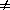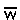Derivation of the Complete Dominance case of the General Selection Model

Given:  genotype:       AA       AB      BB
phenotype:        W0 =   W1W2
or                1    =     11-sq     = [pq] [(q)(W2 - W1) + (p)(W1 - W0)] / []

= [pq] [(q)(1-s) - 1)) + (p)(1 - 1)] / [p2(1) + 2pq(1) + q2(1-s)]

= [pq] [-sq] / [p2 + 2pq + q2 - sq2]q    = -spq2 / (1 - sq2)

Text material © 2004 by Steven M. Carr